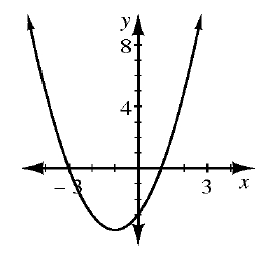### Home > INT2 > Chapter 5 > Lesson 5.2.4 > Problem5-102

5-102.

Write an equation for each representation of a quadratic function given below.

1.Use the $x$-intercepts to help you write the equation.

$y = (x + 3)(x - 1) = x^2 + 2x - 3$

1.  $x$ x column 1 $–4$ x column 2 $–3$ x column 3 $–2$ x column 4 $–1$ x column 5 $0$ x column 6 $1$ x column 7 $2$ x column 8 $3$ x column 9 $4$ $y$ y column 1 $12$ y column 2 $5$ y column 3 $0$ y column 4 $–3$ y column 5 $–4$ y column 6 $–3$ y column 7 $0$ y column 8 $5$ y column 9 $12$

The $x$-intercepts are the points where $y = 0$.

1. Which function has the largest maximum value?

Neither, they both increase indefinitely.